# Homogeneous function

(diff) ← Older revision | Latest revision (diff) | Newer revision → (diff)

of degreeA functionsuch that for all points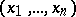in its domain of definition and all real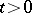, the equationholds, whereis a real number; here it is assumed that for every pointin the domain of, the point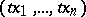also belongs to this domain for any. If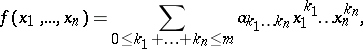that is,is a polynomial of degree not exceeding, thenis a homogeneous function of degreeif and only if all the coefficients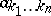are zero for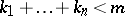. The concept of a homogeneous function can be extended to polynomials invariables over an arbitrary commutative ring with an identity.

Suppose that the domain of definitionoflies in the first quadrant,, and contains the whole ray,, whenever it contains. Thenis homogeneous of degreeif and only if there exists a functionofvariables, defined on the set of points of the form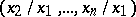where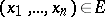, such that for all,If the domain of definitionofis an open set andis continuously differentiable on, then the function is homogeneous of degreeif and only if for allin its domain of definition it satisfies the Euler formula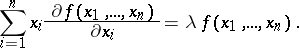How to Cite This Entry:
Homogeneous function. Encyclopedia of Mathematics. URL: http://encyclopediaofmath.org/index.php?title=Homogeneous_function&oldid=11366
This article was adapted from an original article by L.D. Kudryavtsev (originator), which appeared in Encyclopedia of Mathematics - ISBN 1402006098. See original article